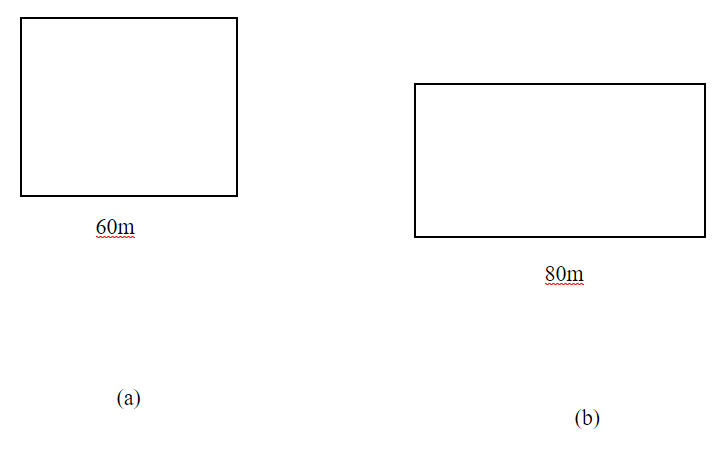Courses
Courses for Kids
Free study material
Free LIVE classes
MoreLIVE
Join Vedantu’s FREE Mastercalss

# A square and a rectangle field with measurement as given in the figure have the same perimeter. Which field has a larger area?Verified
360.9k+ views
Hint: First find the other side of the rectangle by equating the perimeter of square and rectangle. Now use formula for area of square and rectangle to get the final answer.

It is given that perimeter of rectangle = perimeter of square.. (1)
Step 1: Let us suppose that side of square = s
Perimeter of square = $60 + 60 + 60 + 60 = 240$= (4s)
From (I)
$2\left( {l + b} \right) = 240 \\ \Rightarrow 2\left( {80 + b} \right) = 240 \\ \Rightarrow 160 + 2b = 240 \\ \Rightarrow 2b = 240 - 160 \\ \Rightarrow 2b = 80 \\ \Rightarrow b = 40 \\ \\$
So, $l = 80,{\text{ b = 40}}$
Step 2: Now we have to find out whether the area of square has the larger area or the area of rectangle has the larger area:
We know that:
Area of square=$side \times side$
=$60 \times 60 \\ = 3600 \\$
Area of rectangle= $l \times b$
$= 80 \times 40 \\ = 3200 \\$
Therefore, the area of the square is greater than the area of the rectangle that is 3600.

Note: Whenever we face such types of questions, the key concept is that we have to first calculate the perimeter of the square then the perimeter of the rectangle. First, we calculate the perimeter of the square like we did in the question. With the help of the perimeter of the square we will calculate the breadth of the rectangle by applying the formula$2\left( {l + b} \right)$. Second step is to calculate the area of the rectangle and area of square and to check whether the fig a has the larger area or the fig b has the larger area. After applying the formula we get our required answer , like in the above question the area of the square is greater than the area of the rectangle.
Last updated date: 19th Sep 2023
Total views: 360.9k
Views today: 8.60k